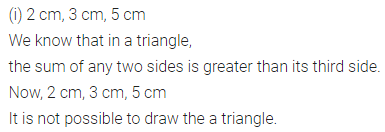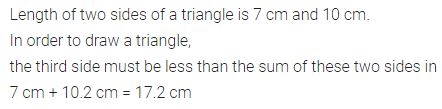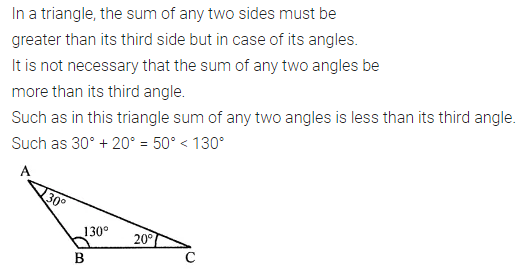ML Aggarwal Class 7 Solutions Chapter 11 Triangles and its Properties Ex 11.4 for ICSE Understanding Mathematics acts as the best resource during your learning and helps you score well in your exams.

## ML Aggarwal Class 7 Solutions for ICSE Maths Chapter 11 Triangles and its Properties Ex 11.4

Question 1.
Is it possible to have a triangle with the following sides?
(i) 2 cm, 3 cm, 5 cm
(ii) 2.5 cm, 4.5 cm, 8 cm
(iii) 10.2 cm, 5.8 cm, 4.5 cm
(iv) 3.4 cm, 4.7 cm, 6.2 cm
Solution:Question 2.
If the lengths of two sides of a triangle are 7 cm and 10 cm, then what can be the length of the third side?
Solution:Question 3.
We know that in a triangle, the sum of lengths of any two sides is greater than the length of the third side. Is the sum of any angles of a triangle also greater than the third angle? If no, draw a rough sketch to show such a case.
Solution: